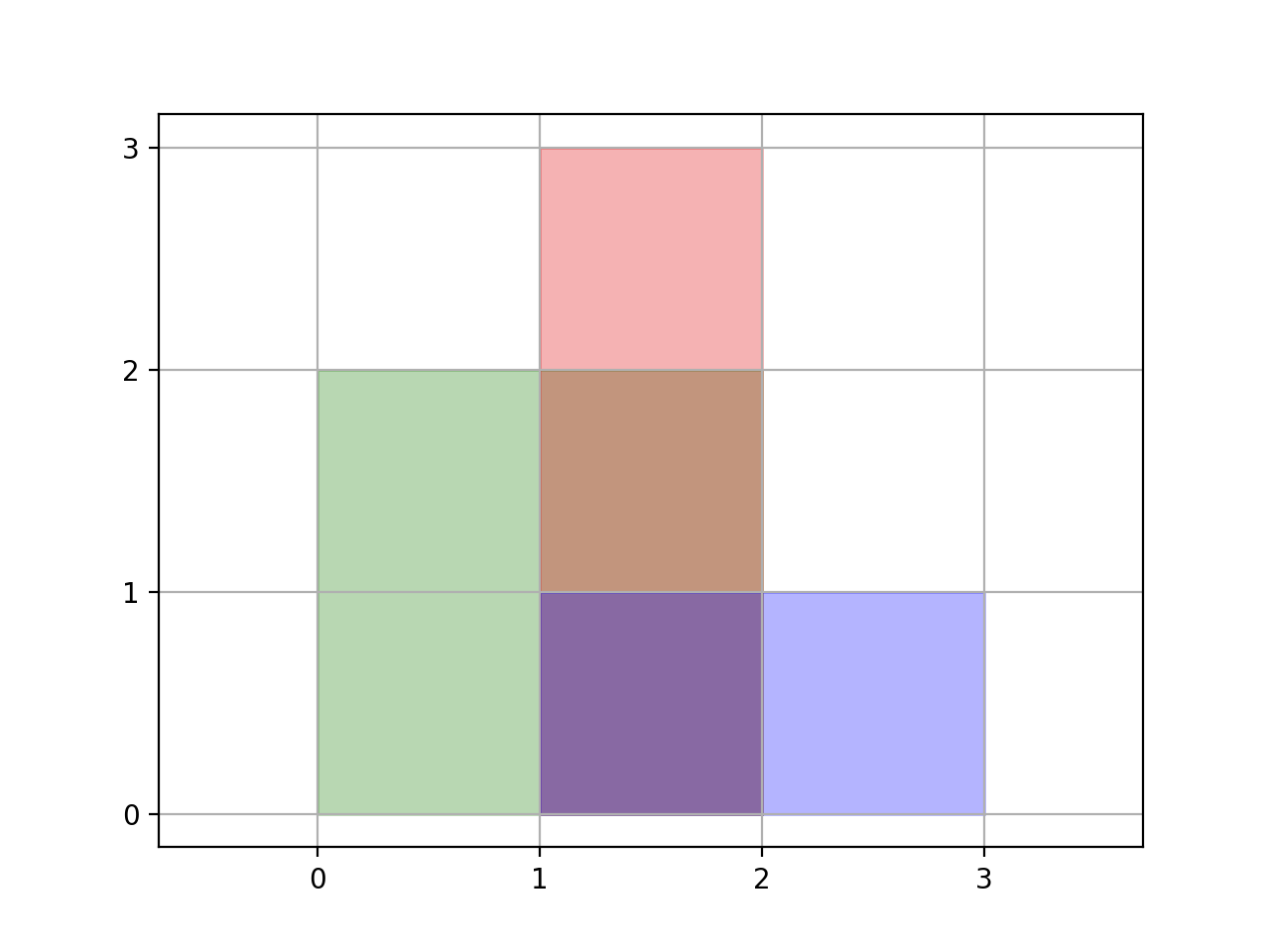## Algorithm

Problem Name: 850. Rectangle Area II

You are given a 2D array of axis-aligned `rectangles`. Each `rectangle[i] = [xi1, yi1, xi2, yi2]` denotes the `ith` rectangle where `(xi1, yi1)` are the coordinates of the bottom-left corner, and `(xi2, yi2)` are the coordinates of the top-right corner.

Calculate the total area covered by all `rectangles` in the plane. Any area covered by two or more rectangles should only be counted once.

Return the total area. Since the answer may be too large, return it modulo `109 + 7`.

Example 1:```Input: rectangles = [[0,0,2,2],[1,0,2,3],[1,0,3,1]]
Output: 6
Explanation: A total area of 6 is covered by all three rectangles, as illustrated in the picture.
From (1,1) to (2,2), the green and red rectangles overlap.
From (1,0) to (2,3), all three rectangles overlap.
```

Example 2:

```Input: rectangles = [[0,0,1000000000,1000000000]]
Output: 49
Explanation: The answer is 1018 modulo (109 + 7), which is 49.
```

Constraints:

• `1 <= rectangles.length <= 200`
• `rectanges[i].length == 4`
• `0 <= xi1, yi1, xi2, yi2 <= 109`

## Code Examples

### #1 Code Example with Javascript Programming

```Code - Javascript Programming```

``````
const rectangleArea = function(rectangles) {
let xnodes = rectangles.reduce((arr, rect) => {
arr.push(rect, rect)
return arr
}, [])
xnodes = [...new Set(xnodes)].sort((a, b) => a - b)
let res = 0n
let overlay
let ynodes
let ysum
for (let i = 1; i < xnodes.length; i++) {
overlay = []
rectangles.forEach(rect => {
if (rect <= xnodes[i - 1] && rect >= xnodes[i]) overlay.push(rect)
})
ynodes = overlay.reduce((set, rect) => {
return set
}, new Set())
ynodes = [...ynodes].sort((a, b) => a - b)
ysum = 0n
for (let i = 1; i < ynodes.length; i++) {
for (let j = 0; j < overlay.length; j++) {
if (overlay[j] <= ynodes[i - 1] && overlay[j] >= ynodes[i]) {
ysum += BigInt(ynodes[i] - ynodes[i - 1])
break
}
}
}
res += ysum * BigInt(xnodes[i] - xnodes[i - 1])
}
return Number(res % BigInt(Math.pow(10, 9) + 7))
}
``````
Copy The Code &

Input

cmd
rectangles = [[0,0,2,2],[1,0,2,3],[1,0,3,1]]

Output

cmd
6

### #2 Code Example with Python Programming

```Code - Python Programming```

``````
class Solution:
def rectangleArea(self, rectangles):
xs = sorted(set([x for x1, y1, x2, y2 in rectangles for x in [x1, x2]] + ))
x_i = {v: i for i, v in enumerate(xs)}
count =  * len(x_i)
L = []
for x1, y1, x2, y2 in rectangles:
L.append([y1, x1, x2, 1])
L.append([y2, x1, x2, -1])
L.sort()
cur_y = cur_x_sum = area = 0
for y, x1, x2, sig in L:
area += (y - cur_y) * cur_x_sum
cur_y = y
for i in range(x_i[x1], x_i[x2]):
count[i] += sig
cur_x_sum = sum(x2 - x1 if c else 0 for x1, x2, c in zip(xs, xs[1:], count))
return area % (10 ** 9 + 7)
``````
Copy The Code &

Input

cmd
rectangles = [[0,0,2,2],[1,0,2,3],[1,0,3,1]]

Output

cmd
6